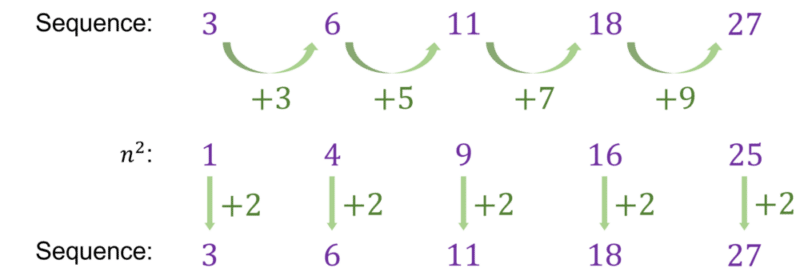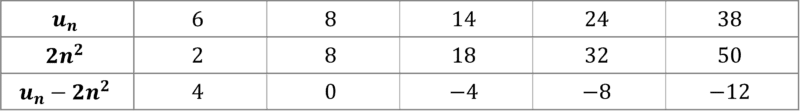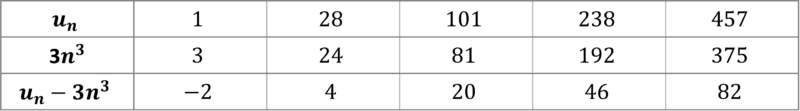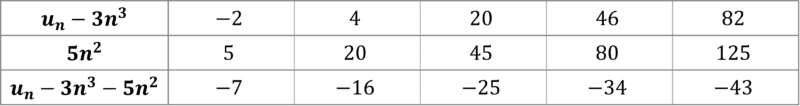# Quadratic, Cubic and Harder Sequences

GCSELevel 4-5Level 6-7Cambridge iGCSE

## Quadratic, Cubic and Harder Sequences

Following on from linear sequences, which have a common difference between each term, the sequences found on this page are a bit more complicated, as you have recognise the difference between the differences.

For quadratic and cubic sequences you will need to work out the second and third difference respectively.

## Simple Quadratic and Cubic Sequences

For certain simpler quadratic and cubic sequences we can find the $n$th term by comparing to the list of square and cubic numbers.

Example:

Find the $\textcolor{#10a6f3}{n}$th term for the quadratic sequence with the following first $5$ terms:

$\textcolor{#10a6f3}{3,6,11,18,27}$

We can see that the differences between each term are not equal.Comparing with the sequence $\textcolor{#10a6f3}{n^2}$, each term in our sequence is $\textcolor{#10a6f3}{2}$ greater than the corresponding terms in the sequence $n^2$.

Therefore, we can conclude that the $n$th term of this sequence is $\textcolor{#10a6f3}{n^2+2}$.

Level 4-5GCSECambridge iGCSE

For harder quadratic sequences you will be required to work out the second difference (or differences between the differences) to work out the coefficient of the $n^2$ term in the $n$th term.

You can then subtract the sequence $\textcolor{#00bfa8}{a}n^2$ (where $\textcolor{#00bfa8}{a}$ is to be found) term by term away from your original sequence, leaving you with a linear sequence to work with.

Example:

Find the $n$th term of the sequence with the following first $5$ terms

$6,8,14,24,38$

Firstly we need to find the differences until they are constant.

In this case we can see that the first differences are $+2,+6,+10,+14$ and then the second difference is $\textcolor{#10a6f3}{+4}$.The coefficient of the $n^2$ term of a quadratic sequence is half of the second difference, which in this case is $4\div2=\textcolor{#00bfa8}{2}$.

We then subtract the sequence with $n$th term $2n^2$ away from our original sequence, $u_n$.This leaves us with a linear sequence, which has a term to term difference of $-4$ and therefore an $n$th term of $\textcolor{#10a6f3}{-4n+8}$.

Thus the $n$th term to the quadratic sequence is $\textcolor{#10a6f3}{2n^2-4n+8}$.

Level 6-7GCSECambridge iGCSE

## Cubic Sequences

To find the $n$th term of a cubic sequence we will need to find the first, second and third differences.

Similar to a quadratic sequence you then subtract $an^3$ from your original sequence, leaving you with a quadratic sequence, where you then follow the same steps as above to find the $n$th term.

Example:

Find the $n$th term of the sequence with the following $5$ terms

$1,28,101,238,457$

We need to first find the differences until they are constant.

In this case we can see that the first differences are $\textcolor{#10a6f3}{+27,+73,+137,+219}$, the second differences are $\textcolor{#10a6f3}{+46,+64,+82}$ and then the third difference is $\textcolor{#10a6f3}{+18}$.The coefficient of the $n^3$ term is $\textcolor{#00bfa8}{\dfrac{k}{6}}$, where $\textcolor{#00bfa8}{k}$ is the constant third difference.

Subtracting $3n^3$ away from the sequence:We are now left with a quadratic sequence with a second constant difference of $+10$.

So the coefficient of $n^2$ is $10\div 2=\textcolor{#00bfa8}{5}$.

Subtracting $5n^2$ away from $u_n-3n^3$.This now leaves with us a linear sequence with an $n$th term of $\textcolor{#10a6f3}{-9n+2}$.

Hence the $n$th term of this cubic sequence is $\textcolor{#10a6f3}{3n^3+5n^2-9n+2}$.

Level 6-7GCSECambridge iGCSE

## Exponential Sequences

Exponential sequences increase by a common ratio from term to term, with the $n$th term being defined as

$\textcolor{#d11149}{a}\times \textcolor{#00d865}{r}^{(n-1)}$

where $\textcolor{#d11149}{a}$ is the first term and $\textcolor{#00d865}{r}$ is the value that you multiply by each time.

Example:

An exponential sequence $x_n$ has the first $5$ terms

$5,15,45,135,405$

Find the $n$th term of the sequence.

To find $\textcolor{#00d865}{r}$, divide the next term by the previous term.

$\textcolor{#00d865}{r}=\dfrac{x_{n+1}}{x_n}=\dfrac{15}{5}=\textcolor{#00d865}{3}$

Therefore the $n$th term is $\textcolor{#d11149}{5}\times \textcolor{#00d865}{3}^{(n-1)}$, as $\textcolor{#d11149}{a=5}$.

Level 6-7GCSECambridge iGCSE

## Quadratic, Cubic and Harder Sequences Example Questions

Comparing with the sequence $n^2$, we can see that each term is $3$ less.

So the $n$th term is $n^2-3$.

Substituting in $n=25$ to find the $25$th term:

$25^2-3=622$

The first differences between terms are $+16,+22,+28,+34$

The second difference between terms is $+6$

Therefore, the coefficient of the $n^2$ term is $6\div 2=3$

Subtracting $3n^2$ away from the sequence term by term gives us the following linear sequence

$6,13, 20,27,34$

The $n$th term of this sequence is $7n-1$

Thus, the $n$th term of the quadratic sequence is $3n^2+7n-1$

To work out the next term in this sequence we need to find the first, second and third differences.

First differences: $+5,+19,+45,+83$

Second differences: $+14,+26,+38$

Third difference: $+12$

Working backwards we know that the next second difference will be $+50$ and therefore the next first difference will be $+133$.

Therefore, the next term in this sequence is $156+133=289$

First differences: $+5,+11,+23,+41$

Second differences: $+6, +12,+18$

Third difference: $+6$

Coefficient of the $n^3$ term $=6\div6=1$

Subtracting $n^3$ away from the sequence leaves us with the following sequence

$0,-2,-10,-24,-44$

The first differences of this quadratic sequence are $-2,-8,-14,-20$ and the second difference is $-6$

Therefore, the $n^2$ coefficient is $-6\div 2=-3$

Subtracting $-3n^2$ away from the quadratic sequence gives us the linear sequence

$3, 10,17,24,31$

The $n$th term of this linear sequence is $7n-4$

Putting everything together gives us the $n$th term of our cubic sequence, which is $n^3-3n^2+7n-4$

a) Work out the common ratio, $r$ from term to term.

$r=\dfrac{12}{3}=4$

Therefore the $n$th term is $3\times 4^{(n-1)}$

b) Substituting $n=10$ gives

$3\times 4^{(10-1)}=786432$

## You May Also Like...### MME Learning Portal

Online exams, practice questions and revision videos for every GCSE level 9-1 topic! No fees, no trial period, just totally free access to the UK’s best GCSE maths revision platform.

£0.00